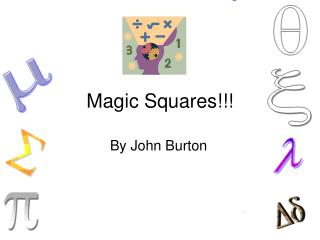DownloadDownload PresentationMagic Squares!!!

# Magic Squares!!!

Download Presentation## Magic Squares!!!

- - - - - - - - - - - - - - - - - - - - - - - - - - - E N D - - - - - - - - - - - - - - - - - - - - - - - - - - -
##### Presentation Transcript

1. Magic Squares!!! By John Burton

2. What Are Magic Squares? A Magic Square is a grid (or matrix) containing numbers from 1 to n (where n is any number), and where every row, column and diagonal adds to the same number The most basic example is the 1 x 1 square, shown below

3. Examples of Magic Squares One of the most famous magic squares is that of Albrecht Dürer. It was created in 1514 and is shown below 16 3 2 13 5 10 11 8 9 6 7 12 4 15 14 1

4. Dürers Square As you can see from the square, the total of each row, column, diagonal and small square is 34. You can also see that the year it was made (1514) appears in the square Here is the year

5. Some More Magic Squares 8 1 6 The total for each row, column etc. is 15 3 5 7 4 9 2 The total for each row, column here is 50

6. How do magic squares work? Magic squares can be made to work in several ways. A 3x3 magic square is made in a different way to a 4x4 magic square For example, magic squares can be constructed in the following way:

7. Constructing magic squares To construct 4 x 4 magic squares, we need the following basic squares: These squares identify where the numbers (these can be any numbers) go

8. Constructing magic squares 2 B1 B2 B3 B4 B5 B6 B7

9. Constructing magic squares 3 From these squares, (any) numbers are chosen and put into the following formula: 9B1+7B2+6B3+7B4-B5+2B6+3B7 The numbers are then put into a magic square, corresponding with were the 1s’ are, in the above basic squares To see the basic squares again, click here

10. Constructing the Dürer magic square Start in the bottom right corner of the grid, and count along, but only put the numbers you count on the diagonal lines. i.e. follow the path marked out below, putting numbers on the diagonals 16 13 10 11 6 7 4 1 As you can see, these numbers lie on the diagonal lines

11. Constructing the Dürer magic square 2 Next, starting at the bottom left, count backwards from 16, putting the numbers in the blank spaces. 16 13 3 2 5 10 11 8 6 7 9 12 4 1 15 14

12. Constructing the Dürer magic square 3 As you can see, the Dürer magic square has now been constructed 16 3 2 13 5 10 11 8 9 6 7 12 4 15 14 1

13. The Magic Formula To find out what the magic total is, we can use a formula, which will tell us the total of the rows, columns, diagonals etc. The formula is ½n(n²+1) Where n is the number of rows

14. Deriving the formula To derive the formula for the magic square, we must first assign the magic total. Let’s call this x. We must then assign the number of rows (i.e. the size of the square). As you may have gathered, this will be called n

15. Deriving the formula 2 We can write this out as 1+2+3+4+…+n²=n.x From Pure maths, this can be written as: n^2 ∑ = n.x i=1

16. Deriving the formula 3 From pure maths, we know that the formula for this series is: n.x= ½n²(n²+1) We then divide both sides by n, to get: x= ½n(n²+1) This formula only works for magic squares, which contain integers (i.e. whole numbers, no decimals)